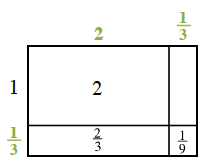Home > CC1 > Chapter Ch8 > Lesson 8.1.1 > Problem8-11

8-11.

Copy and complete each of the generic rectangles below. Be sure to state the final product. Homework Help ✎

1.  $1$ $2$ $\frac{2}{3}$ $\frac{1}{9}$

• Determine which values go on the outside of the rectangle.

Fill in the empty squares inside the rectangle, and add all the numbers in the rectangle. Do not forget to use a common denominator if necessary.$3\frac{1}{9}$

1.  $4$ $8$ $1$ $\frac{1}{8}$

Use the same method as part (a) to find the answer.